Search

About 245 Search Results Matching Types of Worksheet, Worksheet Section, Generator, Generator Section, Subjects matching Math, Grades matching 3rd Grade, Similar to Spring Beginning Letters Worksheet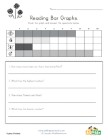Spring Bar Graph Worksheet

Use the bar graph to answer the questions about th...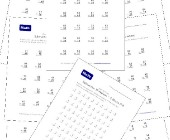Subtraction Worksheets for Kids

This set of free math worksheets is geared towards...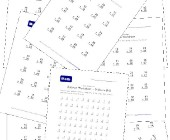This collection of addition worksheets includes si...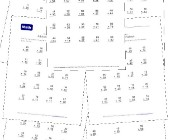Math Help - Addition Worksheets

This group of addition worksheets included a varie...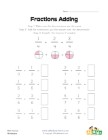Simple Fraction Addition Worksheet

This fractions worksheet has 8 simple fraction add...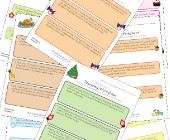Rounding Worksheets

Check out our collection of rounding worksheets in...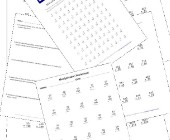Multiplication Worksheets for Kids

This set of math worksheets is geared towards help...